Next: Displacement Operators Up: Position and Momentum Previous: Momentum Representation

# Uncertainty Relation

How is a momentum space wavefunction related to the corresponding coordinate space wavefunction? To answer this question, let us consider the representative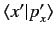of the momentum eigenkets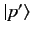in the Schrödinger representation for a system with a single degree of freedom. This representative satisfies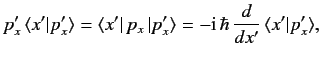(182)

where use has been made of Equation (169) (for the case of a system with one degree of freedom). The solution of the above differential equation is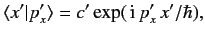(183)

where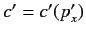. It is easily demonstrated that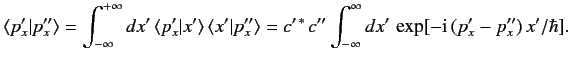(184)

The well-known mathematical result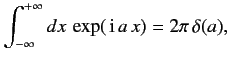(185)

yields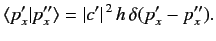(186)

This is consistent with Equation (171), provided that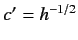. Thus,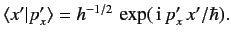(187)

Consider a general state ket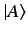whose coordinate wavefunction is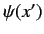, and whose momentum wavefunction is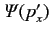. In other words,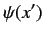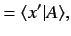(188)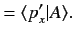(189)

It is easily demonstrated that(190)

and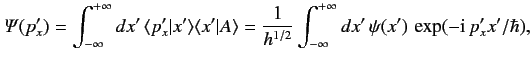(191)

where use has been made of Equations (118), (172), (185), and (187). Clearly, the momentum space wavefunction is the Fourier transform of the coordinate space wavefunction.

Consider a state whose coordinate space wavefunction is a wavepacket. In other words, the wavefunction only has non-negligible amplitude in some spatially localized region of extent. As is well-known, the Fourier transform of a wavepacket fills up a wavenumber band of approximate extent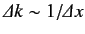. Note that in Equation (190) the role of the wavenumber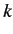is played by the quantity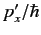. It follows that the momentum space wavefunction corresponding to a wavepacket in coordinate space extends over a range of momenta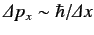. Clearly, a measurement of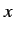is almost certain to give a result lying in a range of width. Likewise, measurement of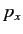is almost certain to yield a result lying in a range of width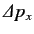. The product of these two uncertainties is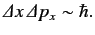(192)

This result is called the Heisenberg uncertainty principle.

Actually, it is possible to write the Heisenberg uncertainty principle more exactly by making use of Equation (83) and the commutation relation (138). We obtain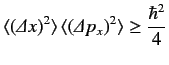(193)

for any general state. It is easily demonstrated that the minimum uncertainty states, for which the equality sign holds in the above relation, correspond to Gaussian wavepackets in both coordinate and momentum space.Next: Displacement Operators Up: Position and Momentum Previous: Momentum Representation
Richard Fitzpatrick 2013-04-08